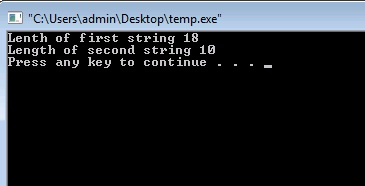# String in C (Standard Library Functions) – Part 4

Also Read: String in C – Part 3

In the last tutorial I gave a brief introduction of the relation between strings and pointers. I also told you about the 2D array of characters. Today I will tell you about some commonly used standard library string functions. These functions are made by the compiler vendors to speed up the manipulation of strings in C programming. So lets get started.

## Standard Library String Functions

There are more than 18 standard library string function but only 4 of them are used very frequently. So I will only discuss those 4 functions which are strlen(), strcpy(), strcat(), strcmp().

Note: The header file that we will use for these functions is string.h.

## strlen() in C

As its name suggest, this function is made to calculate the length of the string. By using this function we can calculate the number of characters in any string.

OutputExplanation
Here you can see I have calculated the length of the string by two ways. So you can either pass string or string variable. Remember while calculating the length it doesn’t count ‘’ character.

## strcpy() in C

This function is used to copy contents of one string to another. To use this function we just have to pass the base address of source and target string. Consider the below example.

Output## strcat() in C

This string function is used to concatenate (combine) two strings. To use this function we have to pass the base address of the two strings. Consider carefully the below program code.

Output## strcmp() in C

Do you remember I told you that we can’t compare two strings directly just like common integer variables. This is because it will always give base address of the string to compare. Strcmp() function is used to compare two strings. This function returns 0 if the strings are identical  and returns the difference of ASCII values when a mismatch occurs. Consider the below program carefully.

OutputExplanation
In the first call I have compared source string with target string that returns -3 which is numeric difference of their mismatch ASCII characters. In the second call, I have passed two identical strings. Therefore 0 is returned by the function.

### You May Also Like:

Category: C Tutorials